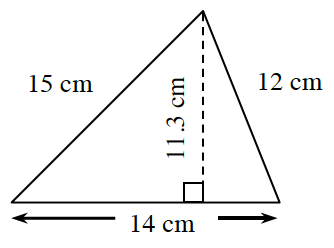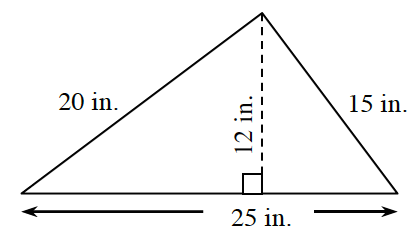### Home > CC1 > Chapter 7 > Lesson 7.1.3 > Problem7-32

7-32.

Find the perimeter and area of each triangle below.

The area of a triangle is equal to ($0.5$)(base)(height).
The perimeter of a triangle is the sum of the lengths of all the sides.

1.The base of this triangle is $14$ cm and the height is
$11.3$ cm.

$\text{Area}=(0.5)(14\text{ cm})(11.3\text{ cm})\\ =79.1\text{ sqcm}^2$
Can you find the perimeter?

1.If someone placed a string around the entire edge of
this triangle, how long would the string have to be?

$\text{ Perimeter} = 25 \text{ in} + 15 \text{ in} + 20 \text{ in} \\ = 60 \text{ inches}$
Can you find the area?